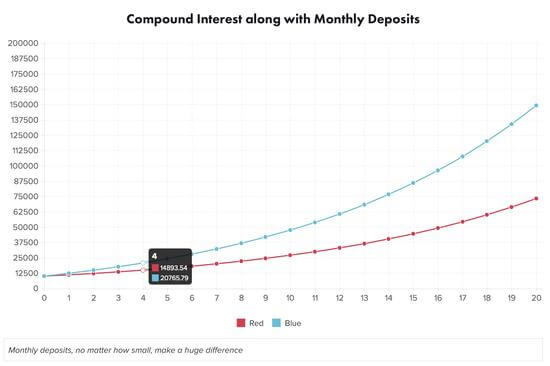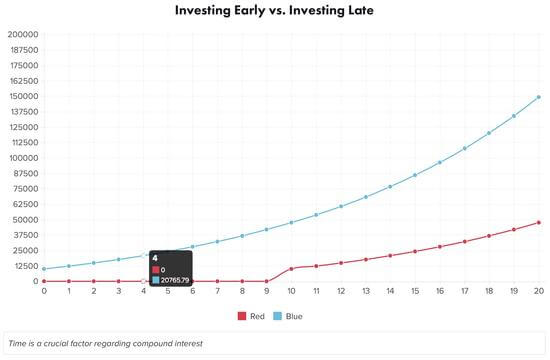GuruFocus

# 複利的神奇魔力

2016 年 6 月 18 日## 討論重點

• 什麼是複利，複利對投資人有多重要？
• 介紹複利的公式和相關的例子
• 複利的神奇魔力

## 什麼是複利？## 複利的分解

FV=P*(1+r/n)^(t*n)

FV=投資的未來價值

P=原始的本金(一開始存入的金額)

r=年利率

t=年數

n=每年複利的次數(若為12，表示每月複利一次；若為1，表示每年複利1次)

FV=10,000*(1+0.15/12)^(10*12)

=10,000*(1.0125)^120

=10,000*(4.44)

=44,402.13

(DEP*(((1+r/n)^(t*n)-1))/(r/n))

DEP=每月存入的金額

r=年利率

t=年數

n=每年複利的次數(若為12，表示每月複利一次；若為1，表示每年1次)

FV=[P*(1+r)^(t*n)]+[DEP*(((1+r/n)^(t*n)-1)/(r/n))]]

FV = [10,000 * (1 + 0.15 / 12) ^ (10 * 12)] + [100 * (((1 + 0.15 / 12) ^ (10 * 12) – 1) / (0.15 / 12))]

= 44,402.13 + [100 * ((1.0125 ^ 120 – 1) / 0.0125)]

= 44,402.13 + [100 * ((4.44 – 1) / 0.0125)]

= 44,402.13 + [100 * (3.44 / 0.0125)]

= 44,402.13 + [100 * 275.22]

= 44,402.13 +27,521.71

= \$71,923.84

## 複利的神奇魔力## 結論

• 愛因斯坦稱複利為“史上最偉大的發現“、“世界上的第八大奇蹟”。
• 要達到複利的效果，有三個元素相當地重要：將獲利再投資、每月定期存入以及時間。
• 從上圖可以看到這些元素能夠讓你的投資在短短的十年就達到二至三倍的成長。(譯者/Ing)

GuruFocus》授權轉載GuruFocus